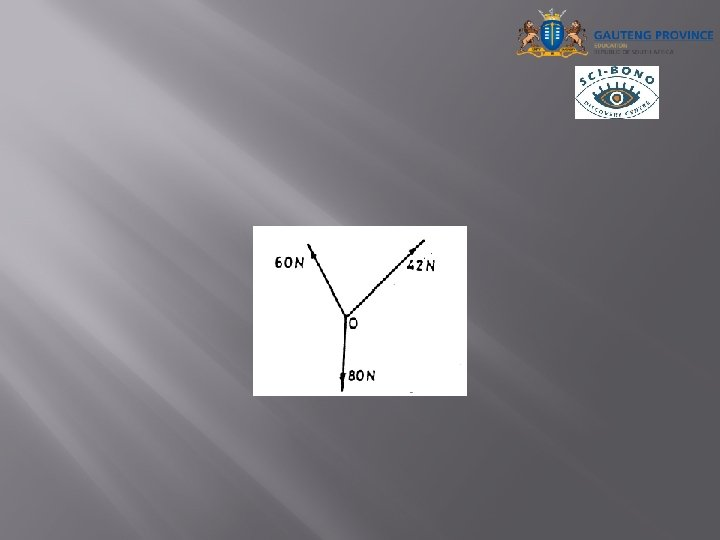# DIFFERENT FORCES BEGINNING PRACTICAL A force which is

• Slides: 19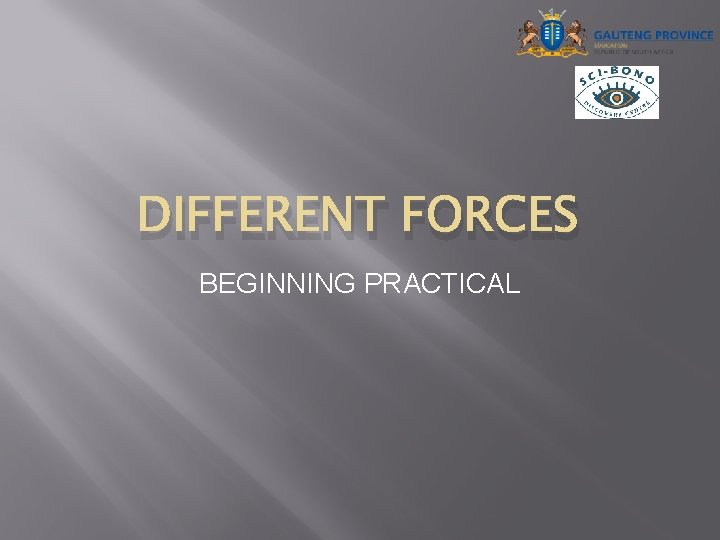DIFFERENT FORCES BEGINNING PRACTICAL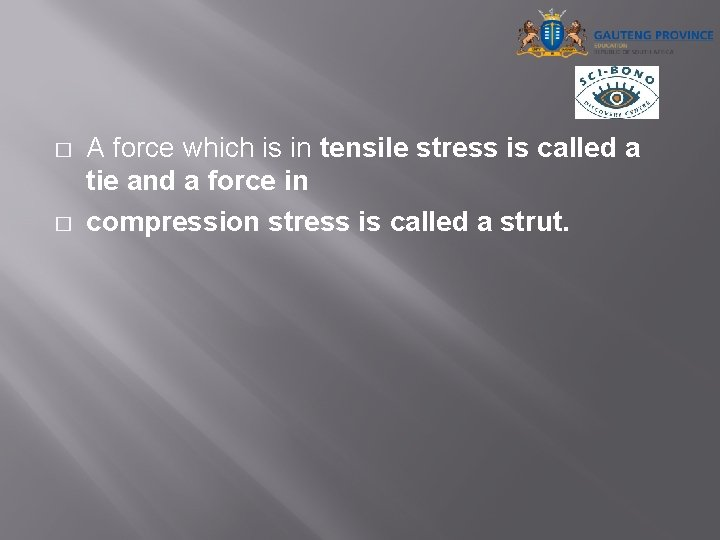� � A force which is in tensile stress is called a tie and a force in compression stress is called a strut.Concept of the triangle of forces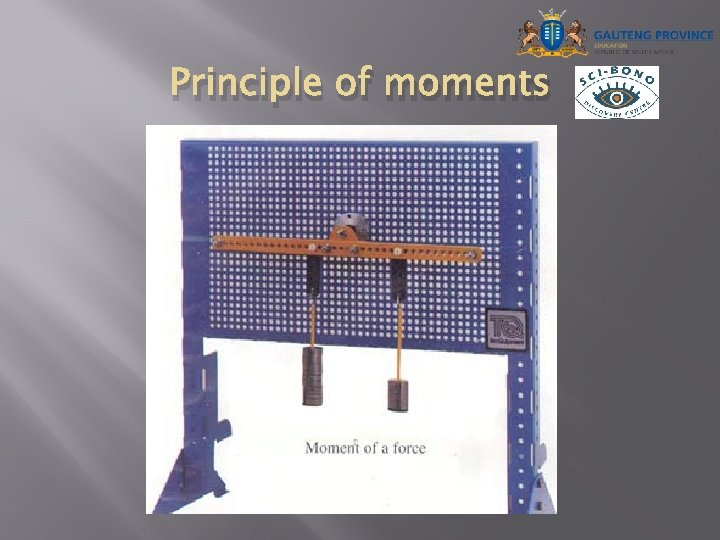Principle of moments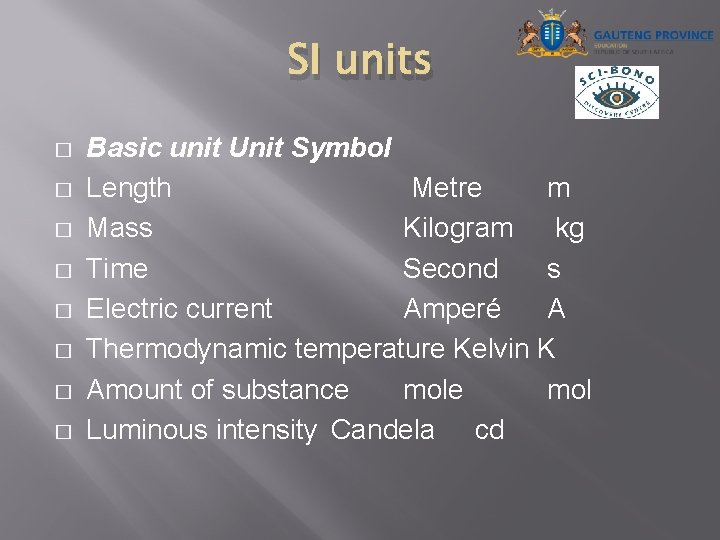SI units � � � � Basic unit Unit Symbol Length Metre m Mass Kilogram kg Time Second s Electric current Amperé A Thermodynamic temperature Kelvin K Amount of substance mol Luminous intensity Candela cd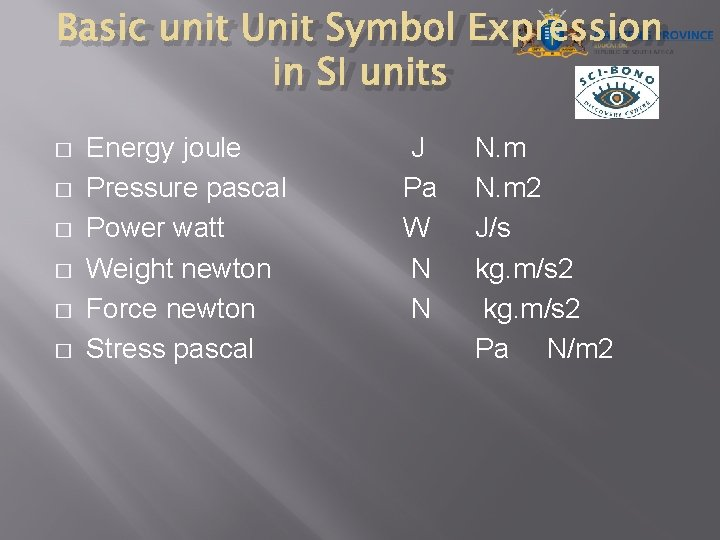Basic unit Unit Symbol Expression in SI units � � � Energy joule Pressure pascal Power watt Weight newton Force newton Stress pascal J Pa W N N N. m 2 J/s kg. m/s 2 Pa N/m 2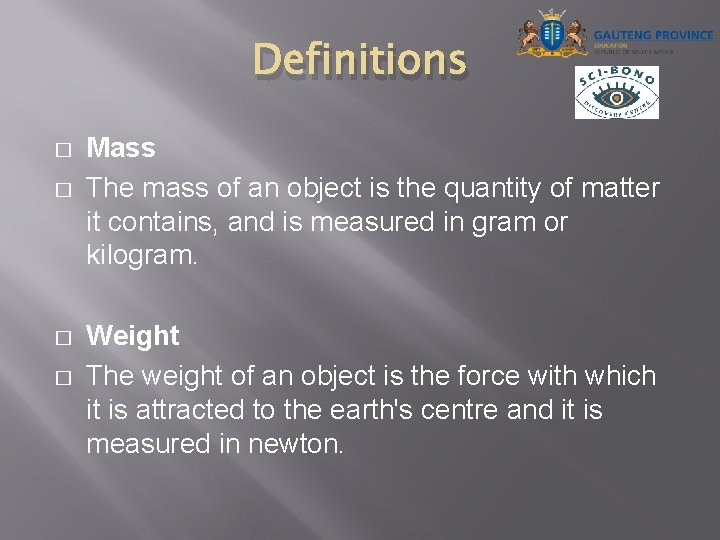Definitions � � Mass The mass of an object is the quantity of matter it contains, and is measured in gram or kilogram. Weight The weight of an object is the force with which it is attracted to the earth's centre and it is measured in newton.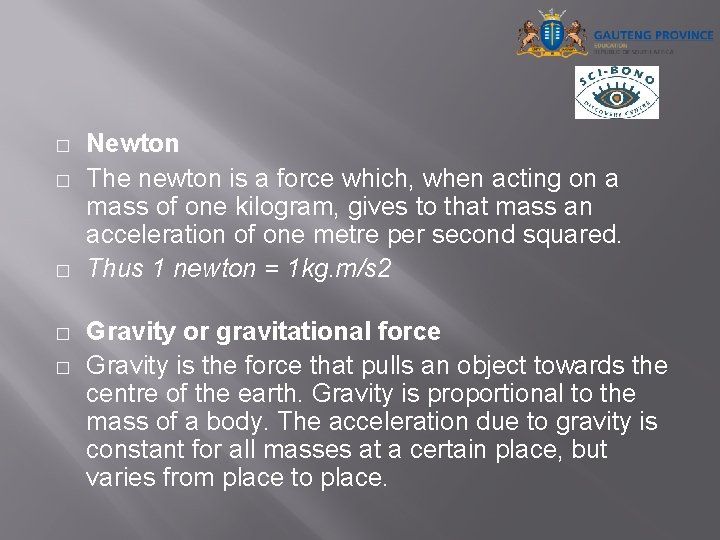� � � Newton The newton is a force which, when acting on a mass of one kilogram, gives to that mass an acceleration of one metre per second squared. Thus 1 newton = 1 kg. m/s 2 Gravity or gravitational force Gravity is the force that pulls an object towards the centre of the earth. Gravity is proportional to the mass of a body. The acceleration due to gravity is constant for all masses at a certain place, but varies from place to place.� � Force A force is that influence which changes or tends to change the state of rest, or uniform motion in a straight line, of an object. If a force is applied to an object, it may have any one, or a combination, of the following: �· Cause a stationary object to move � · Bring a moving object to rest � · Change the direction in which a body is moving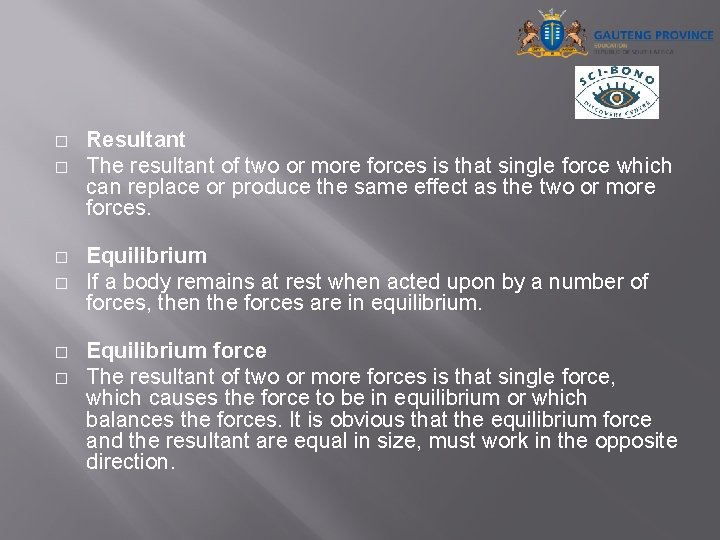� � � Resultant The resultant of two or more forces is that single force which can replace or produce the same effect as the two or more forces. Equilibrium If a body remains at rest when acted upon by a number of forces, then the forces are in equilibrium. Equilibrium force The resultant of two or more forces is that single force, which causes the force to be in equilibrium or which balances the forces. It is obvious that the equilibrium force and the resultant are equal in size, must work in the opposite direction.� � Parallelogram of forces If two pulling forces, acting at a point, are represented in magnitude and direction by two adjacent sides of a parallelogram, the resultant of the two forces is represented in magnitude and direction by the diagonal of the completed parallelogram drawn from the point where the forces act.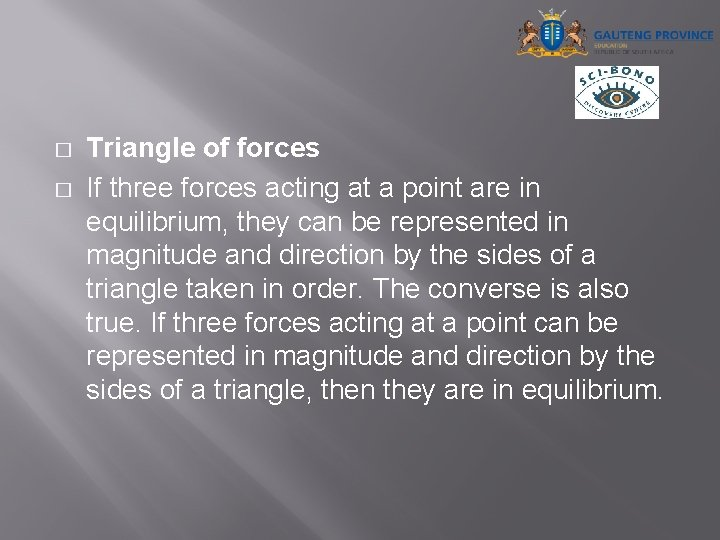� � Triangle of forces If three forces acting at a point are in equilibrium, they can be represented in magnitude and direction by the sides of a triangle taken in order. The converse is also true. If three forces acting at a point can be represented in magnitude and direction by the sides of a triangle, then they are in equilibrium.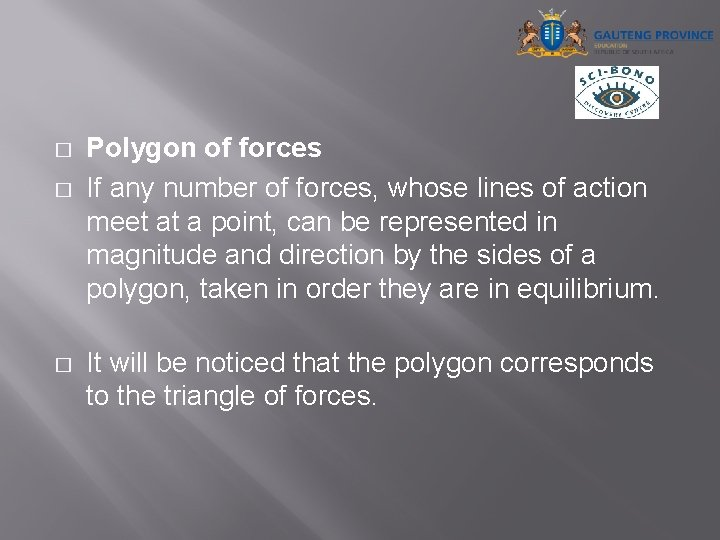� � � Polygon of forces If any number of forces, whose lines of action meet at a point, can be represented in magnitude and direction by the sides of a polygon, taken in order they are in equilibrium. It will be noticed that the polygon corresponds to the triangle of forces.Types of forces � � Tensile force It is the internal force which is present in a material when an external force (p) is applied.� � Compressive force It is the internal force which is present in a material when it offers resistance to an external compressive force (p).� � Shear force It is the internal force present in a material which results in a shear force between two surfaces tending to slide against each other.Features of a force � In order to define a force fully, the following information must be given: �· Point of application � · Magnitude � · Direction � · Nature, that is, whether it pulls or pushes